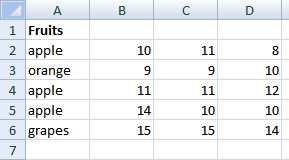Author: Oscar Cronquist Article last updated on January 11, 2019Question:
I'm using excel 2003. This is my problem.Sheet 1 COL A contains fruits, col B to H contains there prizes daily (1 week). take note that in col. A fruits name may randomly repeated in col A. What I need is put in Sheet 2 col A all fruit name but not repeated and put to column B to H, I to N, O to U there prizes .see sample below. hope u understand.
A B C .... I
1 apple 10 11 .... 8
2 orange 9 9 ..... 10
3 apple 11 11 ..... 12
4 apple 14 10 ..... 10
5 grapes 15 15 ..... 14In sheet 2 answer should be like this.
A B C ..... H I J.....N O P.... U
1 apple 10 11 8 11 11 12 14 10 10
2 orange 9 9 10
3 grapes 15 15 14Array formula in cell A2:

=INDEX(Sheet1!\$A\$2:\$A\$6, MATCH(0, COUNTIF(Sheet2!\$A\$1:A1, Sheet1!\$A\$2:\$A\$6), 0))

To enter an array formula, type the formula in a cell then press and hold CTRL + SHIFT simultaneously, now press Enter once. Release all keys.

The formula bar now shows the formula with a beginning and ending curly bracket telling you that you entered the formula successfully. Don't enter the curly brackets yourself.

Copy cell A2 and paste down as far as needed. See this blog post for an explanation: How to extract a unique distinct list from a column

Array formula in cell B2:

=SMALL(IF(\$A2=Sheet1!\$A\$2:\$A\$6, Sheet1!\$B\$2:\$D\$6, ""), COLUMN(A1))

Copy cell B2 and paste B2:K4. Read more about relative and absolute cell references.

Explaining array formula in cell B2

=SMALL(IF(\$A2=Sheet1!\$A\$2:\$A\$6, Sheet1!\$B\$2:\$D\$6, ""), COLUMN(A1))

Step 1 - Filter values in matching rows

The IF function has three arguments, the first one must be a logical expression. If the expression evaluates to TRUE then one thing happens (argument 2) and if FALSE another thing happens (argument 3).

=SMALL(IF(\$A2=Sheet1!\$A\$2:\$A\$6, Sheet1!\$B\$2:\$D\$6, ""), COLUMN(A1))

IF(\$A2=Sheet1!\$A\$2:\$A\$6, Sheet1!\$B\$2:\$D\$6, "")

becomes

IF("apple"={"apple";"orange";"apple";"apple";"grapes"}, {10; 11; 8, 9; 9; 10, 11; 11; 12, 14; 10; 10, 15; 15; 14}, "")

becomes

IF({TRUE;FALSE;TRUE;TRUE;FALSE}, {10; 11; 8, 9; 9; 10, 11; 11; 12, 14; 10; 10, 15; 15; 14}, "")

becomes

{10; 11; 8, ""; ""; "", 11; 11; 12, 14; 10; 10, ""; ""; ""}

Step 2 - Return the k-th smallest value

To be able to return a new value in a cell each I use the SMALL function to filter column numbers from smallest to largest.

=SMALL({10; 11; 8, ""; ""; "", 11; 11; 12, 14; 10; 10, ""; ""; ""}, COLUMN(A1))

becomes

=SMALL({10; 11; 8, ""; ""; "", 11; 11; 12, 14; 10; 10, ""; ""; ""},1)

and returns 8.

* You will also get a weekly newsletter, unsubscribe anytime!
This merging partially works for me, but instead of ordering the values on sheet 2 from smallest to largest, I need to keep the original order for sheet 1, for example instead of:
apple: 8 10 10 10 11 11 11 12 14
keep the original order from sheet 1 like this:
apple: 10 11 8 11 11 12 14 10 10
how can I change the formula(s) to do it?
Thanks!!```Function MergeMatchingRows(SearchValue As Range, SearchRange As Range)
Dim r, c, ic As Single
Dim temp() As Variant
ReDim temp(0)

For r = 1 To SearchRange.Rows.Count
If SearchRange.Cells(r, 1) = SearchValue Then
For c = 2 To SearchRange.Columns.Count
If SearchRange.Cells(r, c) <> "" Then
temp(UBound(temp)) = SearchRange.Cells(r, c)
ReDim Preserve temp(UBound(temp) + 1)
End If
Next c
End If
Next r

ReDim Preserve temp(UBound(temp) - 1)
For c = UBound(temp) To ic
ReDim Preserve temp(UBound(temp) + 1)
temp(UBound(temp)) = ""
Next c

MergeMatchingRows = temp

End Function
```